# Test: Power System Stability of Power Systems

## 10 Questions MCQ Test GATE Electrical Engineering (EE) 2023 Mock Test Series | Test: Power System Stability of Power Systems

Description
Attempt Test: Power System Stability of Power Systems | 10 questions in 30 minutes | Mock test for Electrical Engineering (EE) preparation | Free important questions MCQ to study GATE Electrical Engineering (EE) 2023 Mock Test Series for Electrical Engineering (EE) Exam | Download free PDF with solutions
QUESTION: 1

### A 500-MVA synchronous machine has H1 = 4.6 MJ/MVA, and a 1500 MVA machine has H2 = 3.0 MJ/MVA. The two machines operate in parallel in a power station. The equivalent H constant for the two, relative to a 100 MVA base will be

Solution:

We know that,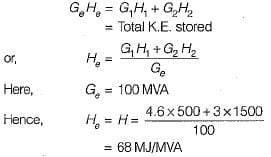QUESTION: 2

Solution:
QUESTION: 3

### Fast acting excitation systems

Solution:

Fast field excitation system is one of the method to improve transient stability of the system.

QUESTION: 4

A shunt reactor in general

Solution:

Shunt reactors are used to reduce the effect of Ferranti effect when a medium or a long line is lightly loaded or is on no-load. It doesn’t affect the steady state power transfer limit.

QUESTION: 5

The angle swings during transients

Solution:
QUESTION: 6

If the inertia constant H = 9.0 MJ/MVA for a 50 MVA generator, the stored energy is

Solution: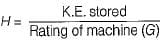or,
K.E. stored = GH = 50 x 9 = 450 MJ

QUESTION: 7

Bundled conductors in a transmission line will

Solution:

Bundled conductors increases self GMD due to which the series inductive reactance is reduced as a result of which power transferred from sending end to the receiving end is increased. Hence, it improves the steady state stability of the system.

QUESTION: 8

A 1500 MVA synchronous generator has an inertia constant of 5.0 MJ/MVA. The inertia constant of the machine relative to a 50 MVA base is

Solution:

Since inertia constant,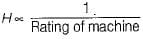∴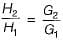or,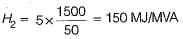QUESTION: 9

If the initial acceleration power of a 100 MVA, 50 Hz synchronous generator having H= 5 MJ/MVA is Xpu, the initial acceleration in elec-deg/s2 and the inertia constant in M J-s/ele-deg respectively will be

Solution:

From swing equation,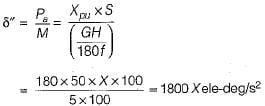Also, inertia constant,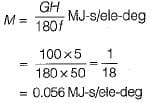QUESTION: 10

The critical clearing angle of a power system is related to

Solution:

tcr and δcr are related to transient stability limit. The fault in a power system must be cleared before δ reaches δcr and before tcr.Use Code STAYHOME200 and get INR 200 additional OFF Use Coupon Code